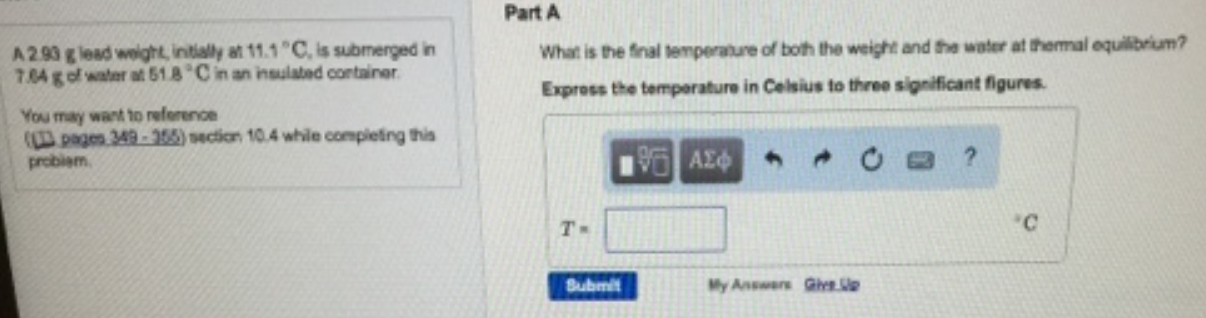Chemistry Calorimetry Solution: A 2.90 g lead weight, initially at 11.1 ºC, is sub...

# Solution: A 2.90 g lead weight, initially at 11.1 ºC, is submerged in 7.64 g of water at 51.8 ºC in an insulated container. What is the final temperature of both the weight and water at thermal equilibrium? Express the temperature in Celsius to three significant figures.

Problem

A 2.90 g lead weight, initially at 11.1 ºC, is submerged in 7.64 g of water at 51.8 ºC in an insulated container. What is the final temperature of both the weight and water at thermal equilibrium? Express the temperature in Celsius to three significant figures.View Complete Written Solution